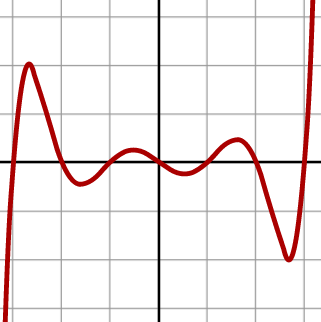# Polynomial phobia abandoned!

Algebra Level 4What is the minimum value of $p(2)$ if the following 4 conditions are followed?

1. $p(x)$ is a polynomial of degree $17$.

2. All roots of $p(x)$ are real.

3. All coefficients are positive.

4. The coefficient of $x^{17}$ is 1.

5. The product of roots of $p(x)$ is -1.

×